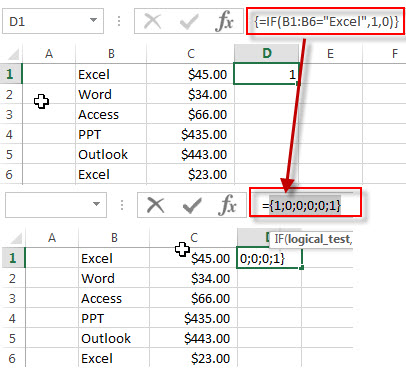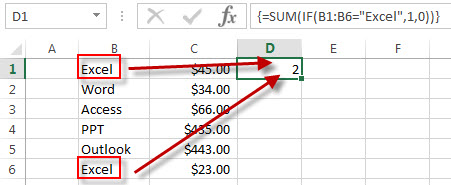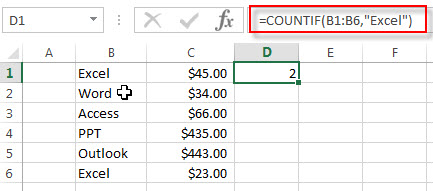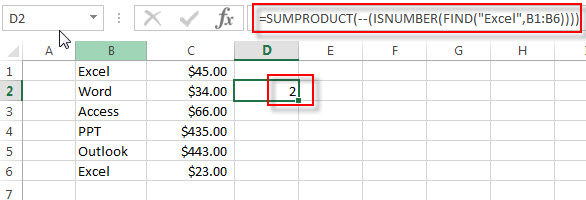# How to Count Cells That Contain Specific Text

This post will discuss that how to count the number of cells that contain specific text or certain text in a specified cells of range in Excel. How to get the total number of cells that contain certain text.

## Count Cells That Contain Specific Text

Assuming that you have a data of list in range B1:B6 that contains some office products and you want to count the number of cells that contain “Excel” in B1:B6. You can use the SUM function in combination with the IF function to create a complex Excel Array Formula as follows:

`{=SUM(IF(B1:B6="Excel",1,0)) }`

Note: when entering this formula into the formula box, you need to press Ctrl+Shift+Enter shortcuts to change this formula as an Array formula.

Let’s see how this formula works:

{=IF(B1:B6=”Excel”,1,0)}This formula will check each cell if it is equal to the specified text string “Excel”, if so, then return value 1. Otherwise, returns value 0.  So this array formula returns another array list like this: {1;0;0;0;0;1}. This array list will pass into the SUM function as its argument.

{=SUM(IF(B1:B6=”Excel”,1,0))}The SUM function will count the values in the array list that returned by the IF function. So it returns value 2.

You can also use another function COUNTIF to achieve the same result. You can use the following formula with COUNTIF function to count the number of cells that contain “Excel” text string in range B1:B6.

`=COUNTIF(B1:B6,"Excel")`The COUNTIF function will count the number of cells in the specified range that contain “Excel” string by matching the content of each cell. And you can also use the wildcard in the criteria argument of COUNTIF.

Another Excel formula can be used to count the number of cells that contain Excel text string, this formula will use the SUMPRODUCT function in combination with the ISNUMBER function and the FIND function as follows:

`=SUMPRODUCT(--(ISNUMBER(FIND("Excel",B1:B6))))`### Related Functions

• Excel SUM function
The Excel SUM function will adds all numbers in a range of cells and returns the sum of these values. You can add individual values, cell references or ranges in excel.The syntax of the SUM function is as below:= SUM(number1,[number2],…)…
• Excel IF function
The Excel IF function perform a logical test to return one value if the condition is TRUE and return another value if the condition is FALSE. The IF function is a build-in function in Microsoft Excel and it is categorized as a Logical Function.The syntax of the IF function is as below:= IF (condition, [true_value], [false_value])….
• Excel COUNTIF function
The Excel COUNTIF function will count the number of cells in a range that meet a given criteria. This function can be used to count the different kinds of cells with number, date, text values, blank, non-blanks, or containing specific characters.etc.= COUNTIF (range, criteria)…
• Excel SUMPRODUCT function
The Excel SUMPRODUCT function multiplies corresponding components in the given one or more arrays or ranges, and returns the sum of those products.The syntax of the SUMPRODUCT function is as below:= SUMPRODUCT (array1,[array2],…)…
• Excel ISNUMBER function
The Excel ISNUMBER function returns TRUE if the value in a cell is a numeric value, otherwise it will return FALSE.The syntax of the ISNUMBER function is as below:= ISNUMBER (value)…
• Excel FIND function
The Excel FIND function returns the position of the first text string (sub string) within another text string.The syntax of the FIND function is as below:= FIND(find_text, within_text,[start_num])…